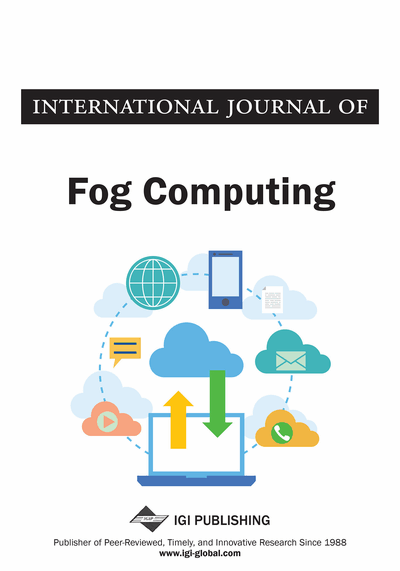# Predictive Modeling for Imbalanced Big Data in SAS Enterprise Miner and R

Son Nguyen (Bryant University, Smithfield, USA), Alan Olinsky (Bryant University, Smithfield, USA), John Quinn (Bryant University, Smithfield, USA) and Phyllis Schumacher (Bryant University, Smithfield, USA)
DOI: 10.4018/IJFC.2018070103

## Abstract

There have been a variety of predictive models capable of handling binary targets, ranging from traditional logistic regression to modern neural networks. However, when the target variable represents a rare event, these models might not be appropriate as they assume that the distribution in the target variable is balanced. In this article, the impact of multiple resampling methods on conventional predictive models is studied. These resampling techniques include the methods of oversampling of the rare events, undersampling of the common events in the data, and synthetic minority over-sampling technique (SMOTE). The predictive models of decision trees, logistic regression and rule induction are applied with SAS Enterprise Miner (EM) software to the revised data. The studied data set is of home mortgage applications which includes a target variable with an occurrence rate of the rare event being 0.8%. The authors varied the percentage of the rare event from the original of 0.8% up to 50% and monitored the associated performances of the three predictive models to see which one worked the best.
Article Preview
Top

## 1. Introduction

In binary classification, when the proportion of one class in the target variable is significantly smaller than the other class (imbalanced data), one faces the problem of classifying a rare event, or imbalanced classification. Traditional classification modeling techniques such as logistic regression and decision trees generally work quite well with a balanced data. However, with a rare event variable, when the data set is very large, and the event of interest is very small, most of these classification methods typically fail to detect the rare event. This can be particularly true when the percentage of the rare event is extremely small, as in the example presented here, where the rare event occurs in less than 1% of the observations. In this situation, all observations are predicted as being in the group consisting of the more dominant or common event.

For example, in the case of a binary target variable, if the rare event makes up only about 1% of the sample, these methods can be correct 99% of the time by predicting all items as falling in the event with the greater probability. In this way, these methods are correct 99% of the time, but may fail to predict the rare event which is most often the aim of the analysis. Unfortunately, many times these rare events, such as fraud, terrorism, etc., are important to predict.

To try to correct this problem, there are possible methods that have been suggested. These methods can be at either the data level, i.e. balancing the data before the modelling stage, or at the algorithmic level, i.e. tuning the existing algorithms to adapt the imbalance of the data.

Regarding the algorithmic approaches, Veropoulos et al. (1999) proposed using two different cost parameters for two classes in the objective functions of support vector machines (SVM) instead of having only one cost parameter, as in the traditional SVM. Imam et al. (2006) suggested modifying the decision boundary to remove the bias toward the majority class. Also modifying the SVM, Wu et al. (2003) used conformally transformed kernels to extend the class boundary region. Several other works on SVM can be found in Batuwita et al. (2013). Maalouf et al. (2016) proposed a method working on logistic regression. The authors used the truncated Newton method in prior correction logistic regression with an added regularization term. Many other modern predictive models have been involved in working with imbalanced data. Wang et al. (2016) proposed using a new loss function in training a deep neural network. Buda et al. (2017) gave an extensive study on the performances of convolutional neural network with imbalanced data.

Along with approaches at the algorithmic level, much research has been conducted at the data level. Methods at this level aim to balance the data before fitting it into conventional classification models. In general, these methods can be divided into two categories: undersampling, i.e. reducing the size of the majority class, and oversampling, i.e. increasing the size of the minority class. Chawla et al (2002) proposed artificially creating observations for the minority class with the idea of linear interpolation. Their method is called Synthetic Minority Over-Sampling Technique (SMOTE). Menardi et al. (2012) proposed ROSE (Random Over-Sampling Examples) where they generated observations for the minority class using its estimated kernel density. Both ROSE and SMOTE are of oversampling method. Although undersampling approaches provide a fast running time for computation, their main drawback is removing a large portion of the data, which may contain important information. The main disadvantages of over-sampling approaches are the slower running time for computation and, in many cases, poor accuracy due to possible noise these methods add to the data.

## Complete Article List

Search this Journal:
Reset
Open Access Articles: Forthcoming
Volume 4: 2 Issues (2021): Forthcoming, Available for Pre-Order
Volume 3: 2 Issues (2020): 1 Released, 1 Forthcoming
Volume 2: 2 Issues (2019)
Volume 1: 2 Issues (2018)
View Complete Journal Contents Listing